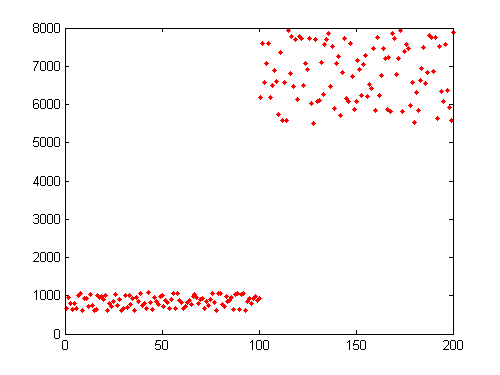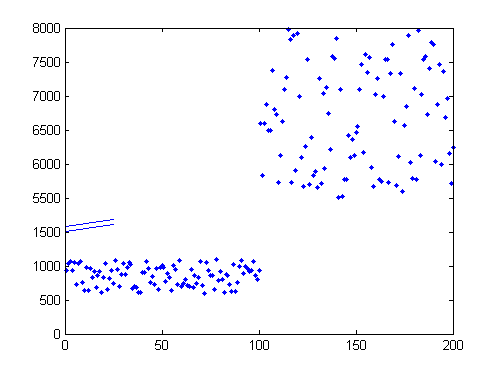# Breaking a plot to improve visualization

### Contents

Suppose you wanted to plot data that spanned two distinct ranges of your dependent variable. Showing all the data on a single plot would waste valuable real estate, and changing to a log scale might obscure some of your data. Classically, you might think of using the SUBPLOT function to create two separate axes to visualize the data. Alternatively, you might want to create a single axes, and to break it to reflect the different ranges. Michael Robbins's BREAKPLOT makes short work of that task. Consider, for example, that you had some sampled engine-speed data collected during two times in the engine's cycle. The first 100 data points are colleced (at sampling times 1:100) while the engine is idling, and the second 100 data are collected (at t = 101:200) while the engine is being revved:
sampleTimes = 1:200;
RPM = [600+rand(1,100)*500, 5500+rand(1,100)*2500];

Note all the wasted "white space" in the traditional plot of these data. Also note how tightly clustered the data can be.
plot(sampleTimes,RPM,'r.')### BREAKPLOT

Now consider how Michael's function allows you to break the y-axis to reflect the range change:
BreakPlot(sampleTimes,RPM,1500,5000,'Line');